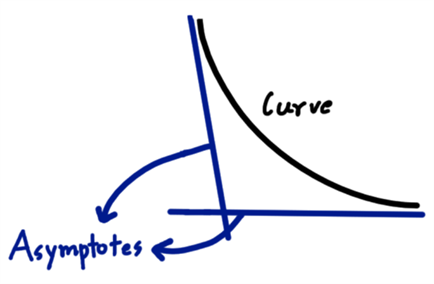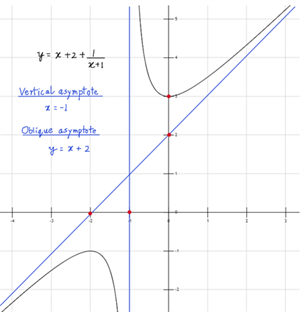# Rational Function & Rational Number

Rational function is the ratio of two polynomial functions where the denominator polynomial is not equal to zero. It is usually represented as R(x) = P(x)/Q(x), where P(x) and Q(x) are polynomial functions. In past grades, we learnt the concept of the rational number. It is the quotient or ratio of two integers, where the denominator is not equal to zero. Hence, the name rational is derived from the word ratio.

## Definition of Rational Function

A number that can be expressed in the form of

$$\begin{array}{l} \frac pq \end{array}$$
where p and q are integers and q ≠ 0, is a rational number.

Just like rational numbers, the rational function definition as:

Definition: A rational function R(x) is the function in the form

$$\begin{array}{l} \frac{ P(x)}{Q(x)}\end{array}$$
where P(x) and Q(x) are polynomial functions and Q(x) is a non-zero polynomial.

R(x) =

$$\begin{array}{l} \frac {P(x)}{Q(x)}\end{array}$$
, Q(x) ≠ 0

From the given condition for Q(x), we can conclude that zeroes of the polynomial function in the denominator do not fall in the domain of the function. When Q(x) = 1, i.e. a constant polynomial function, the rational function becomes a polynomial function.

## Graphing Rational Functions

One very important concept for graphing rational functions is to know about their asymptotes. An asymptote is a line or curve which stupidly approaches the curve forever but yet never touches it. In fig. 1, an example of asymptotes is given.Figure 1: Asymptotes

## Asymptotes of Rational Functions

Rational functions can have 3 types of asymptotes:

1. Horizontal Asymptotes
2. Vertical Asymptotes
3. Oblique Asymptote

### Horizontal Asymptotes

This literally means that the asymptote is horizontal i.e. parallel to the axis of the independent variable. R(x) can only have a horizontal asymptote if

Degree of P(x) ≤ Degree of Q(x)

To determine the asymptotes, divide the numerator and the denominator of R(x) by

$$\begin{array}{l} x^{Degree~of~Q(x)} \end{array}$$
. After that, find the value R(x) approaches as x tends to a very large value. This value gives the height of the asymptote.

### Vertical Asymptotes

R(x) will have vertical asymptotes at the zeros of Q(x). This is because at the zeros of Q(x), Q(x)=0. This means that just towards the left and right of the zero of Q(x), the value of Q will be very small negative and positive number respectively. Value of R(x) will be a largely negative and positive number respectively, towards just left and right of that point.

### Oblique Asymptotes

R(x) will have oblique asymptote if it can be represented in the form

$$\begin{array}{l}T(x)~+~\frac{1}{Q(x)}\end{array}$$
.
When Q(x) ≫ 0, R(x) ≈ T(x). The curve or line T(x) hence becomes an oblique asymptote.

To quote an example, let us take R(x) =

$$\begin{array}{l} \frac{x^2+3x+3}{x+1}\end{array}$$
.

Here, the degree of P(x) is greater than that of Q(x). So, it can’t have a horizontal asymptote. But it will have a vertical asymptote at x=-1. This is because that point is the zero of its denominator polynomial.

It can also be written as R(x) =

$$\begin{array}{l} (x+2)~+~\frac{1}{x+1}\end{array}$$
. So, when x ≫ 0, R(x) ≈ x + 2. So, y = x + 2 will be an oblique asymptote. The graph of the function and all the asymptotes are shown in fig. 2.Figure 2: A rational function with its asymptotes